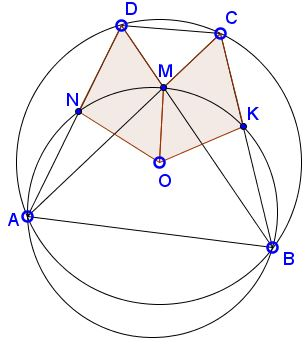# Batman's Problem

### Problem

A quadrilateral $ABCD\;$ is inscribed in a circle with center $O.\;$ Its diagonals meet at $M.\;$ The circumcircle of $ABM\;$ intersect the sides $AD\;$ and $BC\;$ at $N\;$ and $K,\;$ respectively.Prove that the areas of the convex quadrilaterals $NOMD\;$ and $KOMC\;$ are equal.

### Solution

Inscribed angles $CAD\;$ and $CBD\;$ are equal, implying that $\angle MAN=\angle KBM\;$ which, as inscribed into $(AMB),\;$ are then subtended by equal chords: $KM=MN.\;$In addition, $NM\;$ is an antiparallel in $\Delta ABD,$ so that $MN\perp OD.\;$ Similarly, $KM\perp OC.\;$ If $[F]\;$ designates the area of shape $F\;$ then

$[NOMD]=\frac{1}{2}OD\cdot MN=\frac{1}{2}OC\cdot KM=[KOMC].$

### Acknowledgment

The problem that is due to Emil Stoyanov who posted it at the CutTheKnotMath facebook page. The problem has been offered earlier at the XII Zhautykov Olympiad.

•